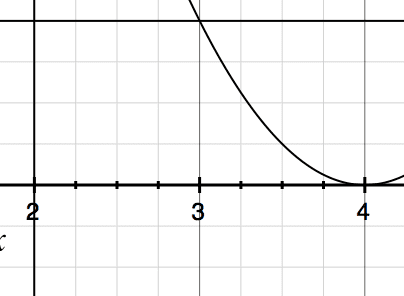# Computer the Volume of a region bounded by 3 curves

Gold Member

## Homework Statement

Let R be the region in the first quadrant bounded by all three of the curves x = 2, y = 1, and y = (x−4)^2.
Compute the volumes V1, V2, and V3 of the solids of revolution obtained by revolving R about the x-axis, the y-axis, and the x = 5 line, respectively.

FIRST, I am trying to conceptualize this problem. I have the 3 necessary curves graphed. A 'triangle' looking figure is formed between y=1 to 4 and x =2 to 3. Do I need to find the volume of THIS figure revolved around the various axis points OR do I need to find the volume between y=0 to 1 and x = 2 to 4.

Both areas are bounded by all 3 curves. My intuition tells me to take the volume of the 'triangle' looking figure, but I did not want to proceed until I figured this part out.

Gold Member
**FIRST POST AND THIS POST ARE TWO SEPARATE PROBLEMS**

The initial problem (previous problem) states:

Let R be the region in the first quadrant bounded by y = 1 − x2. Compute
the volume V of the solid of revolution generated by revolving R about the x-axis by using
(a) slices
and
(b) shells.
Please verify that you obtain the same value of the volume V by method (a) as by method (b).
Use the calculation above to find the volume of the ball of radius 1 (in 3-dimensional space).

Referring to the bold statements, wouldn't the volume of the radius 1 ball be equivalent to the volume of the region (what I had calculated for that problem) since the bounds are from 0 to 1?

LCKurtz
Homework Helper
Gold Member

## Homework Statement

Let R be the region in the first quadrant bounded by all three of the curves x = 2, y = 1, and y = (x−4)^2.
Compute the volumes V1, V2, and V3 of the solids of revolution obtained by revolving R about the x-axis, the y-axis, and the x = 5 line, respectively.

FIRST, I am trying to conceptualize this problem. I have the 3 necessary curves graphed. A 'triangle' looking figure is formed between y=1 to 4 and x =2 to 3. Do I need to find the volume of THIS figure revolved around the various axis points

Yes.

OR do I need to find the volume between y=0 to 1 and x = 2 to 4.

Both areas are bounded by all 3 curves. My intuition tells me to take the volume of the 'triangle' looking figure, but I did not want to proceed until I figured this part out.

Your first thought is correct. ##y=0## is not given as a boundary so your second interpretation is wrong.

•1 person
LCKurtz
Homework Helper
Gold Member
**FIRST POST AND THIS POST ARE TWO SEPARATE PROBLEMS**

You should start a new thread with a new problem.

STEMucator
Homework HelperThe only region that is bounded by all three curves is the one depicted above.

If you rotate this region about the x-axis, what is the volume element ##dV## you would choose to integrate?

I believe choosing vertical slices will produce two integrals. Horizontal slices appear to be better as you will require only one integral.

•1 person
LCKurtz
Homework Helper
Gold Member